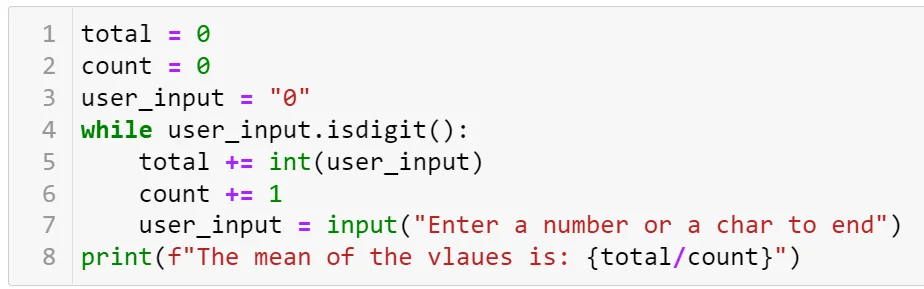31 Mar 2022

Posted on:

31 Mar 2022

0

# Resolved:Question 5, my code should be prone to division by 0 error, but when executed it outputs 0.0 instead

For question 5 i used the following code, which stops when the user input is not numeric and when I tried no inputting any numbers it didn't result in a division by 0 error and I'd like to know why:

total = 0
count = 0
user_input = "0"
while user_input.isdigit():
total += int(user_input)
count += 1
user_input = input("Enter a number or a char to end")
print(f"The mean of the vlaues is: {total/count}")

Instructor
Posted on:

31 Mar 2022

0In lines 1, 2, and 3, the variables `total` and `count` are set to 0, while `user_input` is set to the string "0". In line 4, you check whether `user_input` is a digit. It is, so you enter the loop. In line 5, you increment `total` by `user_input`, so that `total` becomes 0 + 0 = 0. In line 6, you increment the count by 1, so the `count` variable becomes 0 + 1 = 1. Only after that, does the message appear on the screen. Entering nothing in the field (which is equivalent to entering a new line) means that you exit the loop. You then print out `total/count` which is, in fact, 0 / 1 = 0. No error encountered :)